# How to Count Numbers that begin with Specific Value  in Excel

This post will guide you how to count numbers that begin with a specific value or number in Excel 2013/2016 or Excel office 365. How do I count the number of values that begin with a given specific value by using the SUMPRODUCT function and LEFT function in Excel. You can also use the COUNTIF function to count cells that begin with certain characters in Excel.

Assuming that you have a data of list in range B2:B6, and you want to get how many cells in your list which begins with a given number. For example, you wish to calculate the count of number in list where the values begin with “2020”.

## Method1: COUNT Numbers that Begin with Specific Value

To count numbers in your range of list that begin with specific numbers, and you can use a formula based on the SUMPRODUCT function and LEFT function.

=SUMPRODUCT(–(LEFT(B2:B6,4)=”2020″))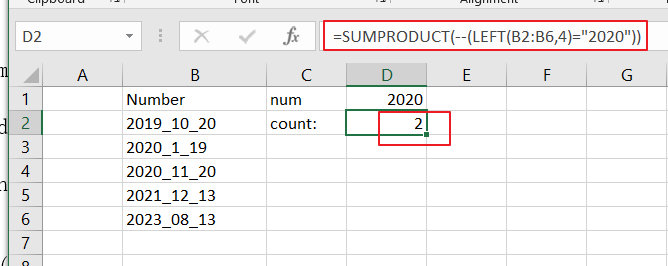Let’s See That How This Formula Works:

=LEFT(B2:B6,4)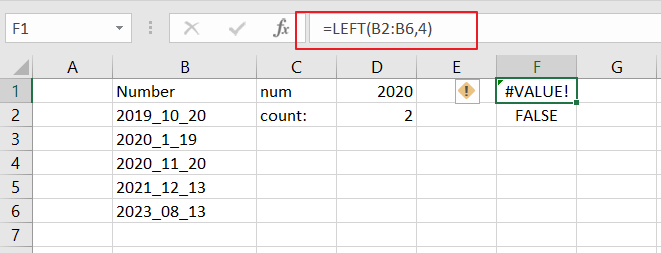This formula will extract the first 4 characters from the given range of cells. And it returns an array result like:

{“2019″;”2020″;”2020″;”2021″;”2023”}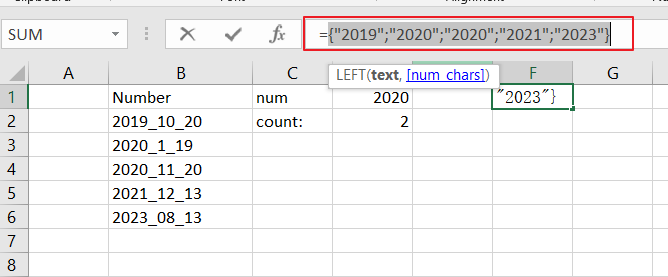=LEFT(B2:B6,4)=”2020″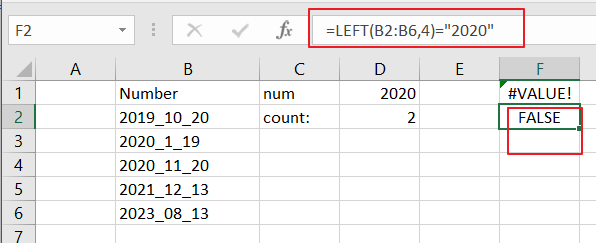The above array result will compare each value to “`2020`” to generate a TRUE or FALSE result. And this formula also returned as an array result of TRUE and FALSE values. Like this:

{FALSE;TRUE;TRUE;FALSE;FALSE}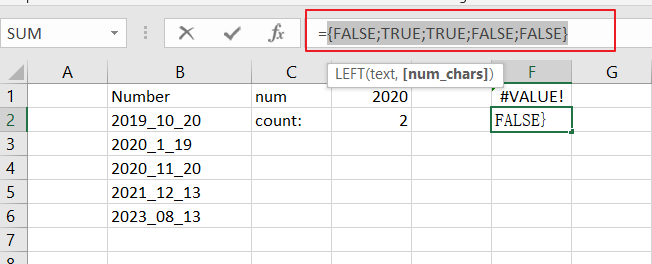=–(LEFT(B2:B6,4)=”2020″)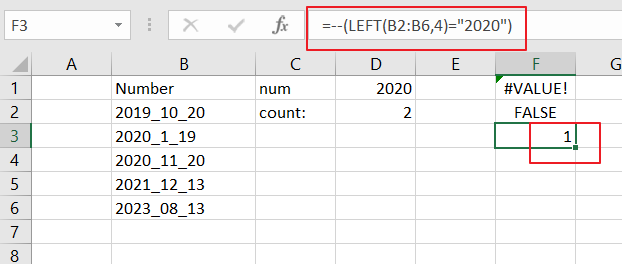Then you need to use a double negative operator to convert TRUE or FALSE values to 1 and 0. It also returned an array result like:

{0;1;1;0;0}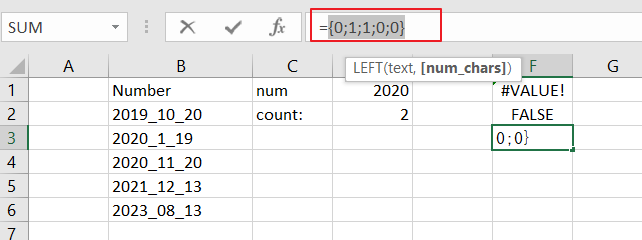SUMPRODUCT function sums the numbers in the above array and returns 2.

## Method2: COUNT Numbers that Begin with Specific Value Using COUNTIF Function

You can also use the COUNTIF function to count the numbers that begin with a particular value. Like below:

=COUNTIF(B2:B6,”2020*”)

This formula use the asterisk wildcard to match any number of uncertain characters in length in your given range of cells.

#1 select Cell D3

#2 enter the above formula into Cell D3

Then you need to press Enter key to apply this formula.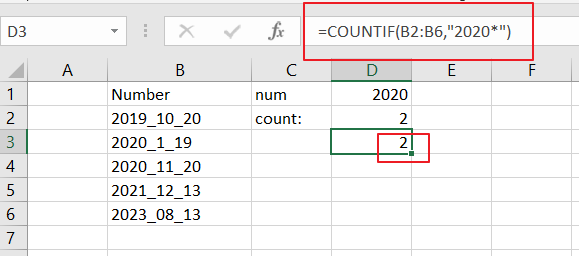### Related Functions

• Excel SUMPRODUCT function
The Excel SUMPRODUCT function multiplies corresponding components in the given one or more arrays or ranges, and returns the sum of those products. The syntax of the SUMPRODUCT function is as below:= SUMPRODUCT (array1,[array2],…)…
• Excel COUNTIF function
The Excel COUNTIF function will count the number of cells in a range that meet a given criteria. This function can be used to count the different kinds of cells with number, date, text values, blank, non-blanks, or containing specific characters.etc.= COUNTIF (range, criteria)…
• Excel LEFT function
The Excel LEFT function returns a substring (a specified number of the characters) from a text string, starting from the leftmost character.The LEFT function is a build-in function in Microsoft Excel and it is categorized as a Text Function.The syntax of the LEFT function is as below:= LEFT(text,[num_chars])…t)…